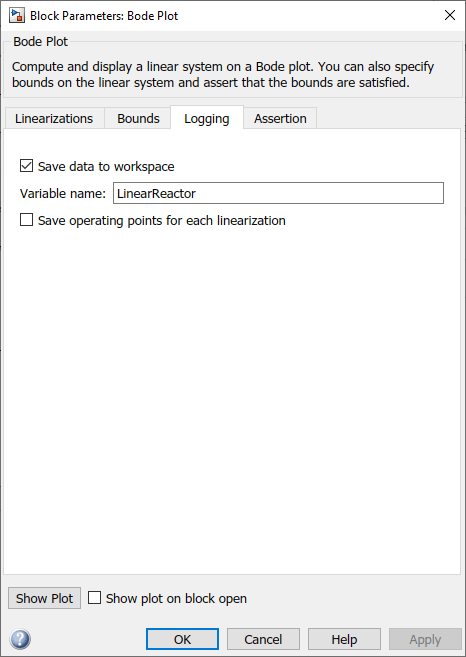# Plot Linear System Characteristics of a Chemical Reactor

This example shows how to plot the linearization of a Simulink® model at particular conditions during simulation. Simulink Control Design™ software provides blocks that you can add to Simulink models to compute and plot linear systems during simulation. In this example, you compute and plot a linearized model for a continuously stirred chemical reactor. Specifically, you linearize the reactor model as it transitions through different operating points.

### Chemical Reactor Model

Open the Simulink model of the chemical reactor.

```open_system('scdcstr') ```The reactor has three inputs and two outputs.

• The `FeedCon0`, `FeedTemp0`, and `Coolant Temp` blocks model the feed concentration, feed temperature, and coolant temperature inputs, respectively.

• The `T` and `CA` ports of the `CSTR` block model the reactor temperature and residual concentration outputs, respectively.

This example focuses on the response from coolant temperature, `Coolant Temp`, to residual concentration, `CA`, when the feed concentration and feed temperature are constant.

### Plot the Reactor Linear Response

The reactor model contains a Bode Plot block from the Simulink Control Design Linear Analysis Plots library. The block is configured with:

• A linearization input at the coolant temperature `Coolant Temp`

• A linearization output at the residual concentration `CA`

The Bode Plot block is configured to perform linearizations on the rising edges of an external trigger signal. The Linearization trigger signal block computes the trigger signal and produces a rising edge when the residual concentration satisfies any one of the following conditions.

• At a steady state value of 2

• In a narrow range around 5

• At a steady state value of 9

To view the Bode Plot block configuration, double-click the block.To view the Bode plot for the block during the simulation, you must open the plot window before simulating the model. To do so, click Show Plot.

Simulate the model.

```sim('scdcstr') ```The Bode plot shows the linearized reactor at three operating points corresponding to the trigger signals generated by the Linearization trigger signal block.

• At 5 sec, the linearization is for a low residual concentration.

• At 38 sec, the linearization is for a high residual concentration.

• At 27 sec, the linearization is as the reactor transitions from a low to high residual concentration.

The linearizations at low and high residual concentrations are similar, but the linearization during the transition has a significantly different DC gain and phase characteristics. At low frequencies, the phase differs by 180 degrees, indicating the presence of either an unstable pole or zero.

### Log the Reactor Linear Response

You can save the computed linear systems to the model workspace. To do so, use the parameters on the Logging tab of the block.

For this example, the Bode Plot block is configured to save the computed responses in the MATLAB® workspace as a `LinearReactor` structure.```LinearReactor ```
```LinearReactor = struct with fields: time: [3x1 double] values: [1x1x3x1 ss] blockName: 'scdcstr/Bode Plot' ```

In this structure, the `values` field stores the linear systems as an array of LTI state-space models. For more information, see Model Arrays. The corresponding simulation times for the linearizations are logged in the `time` field.

Obtain the computed linear models.

```P1 = LinearReactor.values(:,:,1); P2 = LinearReactor.values(:,:,2); P3 = LinearReactor.values(:,:,3); ```

The Bode plot of the linear system at a time of 27 seconds, when the reactor transitions from low to high residual concentration, indicates that the system could be unstable. To confirm this result, displaying the linear systems in zero-pole-gain format.

```zpk(P1) zpk(P2) zpk(P3) ```
```ans = From input "Coolant Temp" to output "CSTR/2": -0.1028 ---------------------- (s^2 + 2.215s + 2.415) Continuous-time zero/pole/gain model. ans = From input "Coolant Temp" to output "CSTR/2": -0.07514 --------------------- (s+0.7567) (s-0.3484) Continuous-time zero/pole/gain model. ans = From input "Coolant Temp" to output "CSTR/2": -0.020462 --------------------- (s+0.8542) (s+0.7528) Continuous-time zero/pole/gain model. ```

```bdclose('scdcstr') ```

### Reference

 Seborg, Dale, Thomas Edgar, and Duncan Mellichamp. Process Dynamics and Control, Second Edition. John Wiley & Sons, Ltd, 2006.